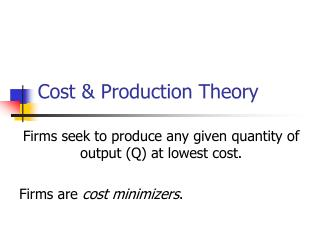Download PresentationCost & Production Theory

Cost & Production Theory - PowerPoint PPT Presentation

Cost & Production Theory. Firms seek to produce any given quantity of output (Q) at lowest cost. Firms are cost minimizers. Costs. C = rK + wL r is the price of capital, w is the wage Cost is the sum of each input quantity multiplied by its price when input prices reflect all costsI am the owner, or an agent authorized to act on behalf of the owner, of the copyrighted work described.
Download PresentationCost & Production Theory

Download Policy: Content on the Website is provided to you AS IS for your information and personal use and may not be sold / licensed / shared on other websites without getting consent from its author.While downloading, if for some reason you are not able to download a presentation, the publisher may have deleted the file from their server.

- - - - - - - - - - - - - - - - - - - - - - - - - - E N D - - - - - - - - - - - - - - - - - - - - - - - - - -
Presentation Transcript
1. Cost & Production Theory Firms seek to produce any given quantity of output (Q) at lowest cost. Firms are cost minimizers.

2. Costs • C = rK + wL • r is the price of capital, w is the wage • Cost is the sum of each input quantity multiplied by its price • when input prices reflect all costs • including opportunity costs

3. Economic Costs are Opportunity Costs • Economic Cost includes both implicit and explicit costs • Explicit Costs – payment to others • Implicit Costs – cost of owned inputs, or other costs that do not generate explicit payments

4. Costs and Output • Long-Run Total Cost or LTC • Combinations of Cost and Output Q • (C1,Q1), (C2,Q2), (C3,Q3) • Long-run average cost • LAC = (LTC/Q) • Long-run marginal cost • LMC = ΔLTC/ΔQ

5. Short-run vs. Long-run • Long-run: all inputs variable • Short-run: one or more inputs fixed • Total Product: Q = f(K0,L) • Average Product of Labor: APL = Q/L • Marginal Product of Labor: MPL = ΔQ/ΔL • Law of diminishing marginal product • As more labor is employed with a fixed amount of capital, labor’s marginal product (MPL) eventually declines

6. Watch the video • Microeconomics The Law of Diminishing Returns: Econ Concepts in 60 Seconds http://youtu.be/M7rA4VfvdAw

7. Short-run Costs • Total Cost = rK0 + wL = TC • Total Fixed Cost = rK0 = TFC • Total Variable Cost = wL = TVC • TC = TFC + TVC • Average Fixed Cost: AFC = TFC/Q • Average Variable Cost: AVC = TVC/Q • Average Total Cost: ATC = TC/Q

8. Short-run Marginal Cost • SMC = ΔTC/ΔQ • ΔTFC/ΔQ = 0 • SMC = ΔTVC/ΔQ = ΔTC/ΔQ • SMC = AVC at its minimum • SMC = ATC at its minimum

9. Watch the videos • Episode 23: Cost Curves http://youtu.be/UI-LL8-dVAs • Cost Curves MC, ATC, AVC, and AFC: Econ Concepts in 60 Seconds http://youtu.be/S3iLMfm6CGY

10. Short-run Cost & Product • AVC and MC are inversely related to APL and MPL • MPL > APL implies MC < AVC • Max MPL corresponds to Min MC • MPL = APL implies MC = AVC • MPL < APL implies MC > AVC

11. Short-run and Long-run Cost • Short-run and long-run costs are equal ONLY at a long-run optimum • The quantity where short-run fixed K0 minimizes long run cost • ATC = LAC • Only at the minimum of LAC are all average and marginal costs equal • LAC = LMC = ATC = SMC

12. Watch the video • 9.2 - Long-Run Cost Structure http://youtu.be/8I6BIuCGuaE

13. Economies of Scale • Economies of scale: LAC is decreasing • Costs increase less than proportionately with output • Diseconomies of scale: LAC increasing • Costs increase more than proportionately with output • Constant returns to scale: LAC = LMC • Costs increase exactly in proportion to output • Minimum Efficient Scale or MES • The quantity at which economies of scale end and constant returns begin

14. Watch the video • Economies and Diseconomies of Scale.mp4 http://youtu.be/6TW-o1NqV0I

15. Economies of Scope • A firm can produce two products together more cheaply than producing each product separately, or • C(X,Y) < C(X) + C(Y)